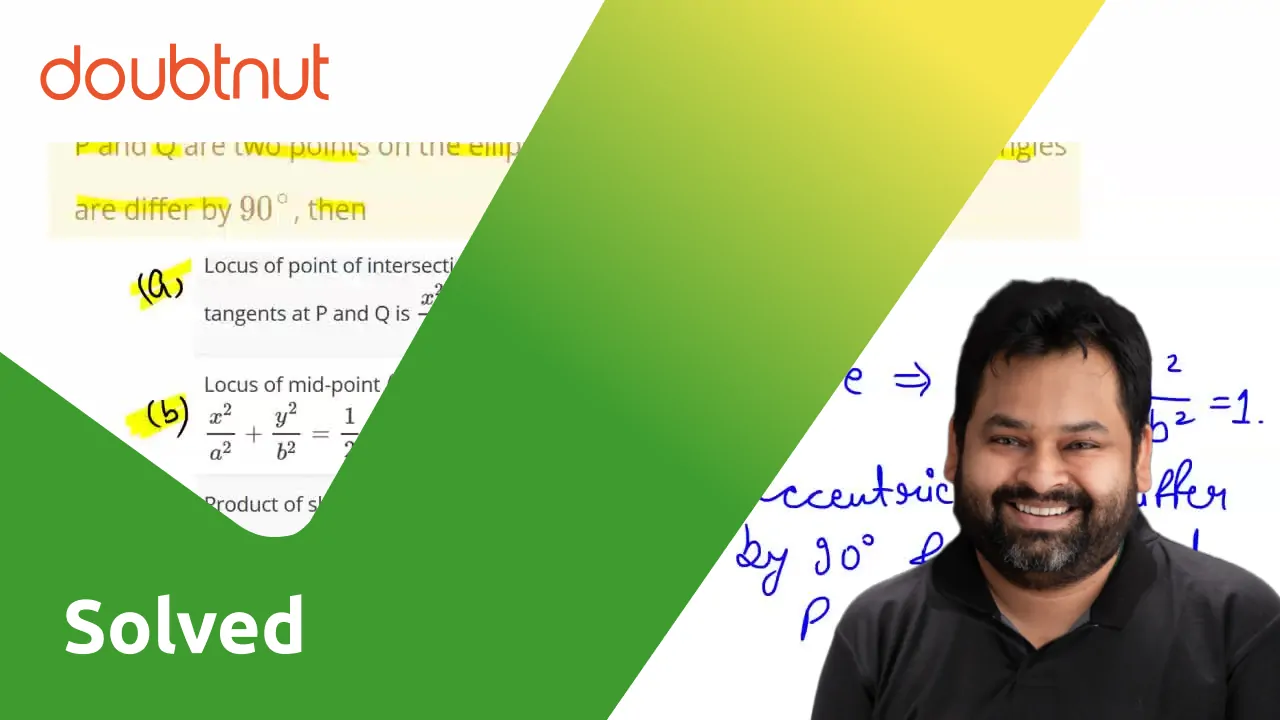Doubtnut App - Get 50 Lakh+ video solutions better than GoogleInstall Now

# P and Q are two points on the ellipse x2a2+y2b2=1 whose eccentric angles are differ by 90∘, then

A

Locus of point of intersection of tangents at P and Q is x2a2+y2b2=2

B

Locus of mid-point (P,Q) is x2a2+y2b2=12

C

Product of slopes of OP and OQ ehere O is the centre is b2a2

D

Max. area of ΔOPQ is 12ab

Text Solution
Verified by Experts

## P=(acosθ,bsinθ) and Q=(−asinθ,bcosθ) Tangent at P,xcosθa+ysinθb=1 (1) −xsinθa+ycosθb=1 (2) Elimination θ, by squaring and adding (1)2+(2)2⇒x2a2+y2b2=2, which is required locus. ∴ (a) is correct Now mid (PQ)=(a(cosθ−sinθ)2b(sinθ+cosθ)2)=(x,y) cosθ−sinθ=2xa (3) cosθ+sinθ=2yb (4) Squaring and adding (3) and (4), we get locus as x2a2+y2b2=12 ∴ (b) is correct Slope of OP=bsinθacosθ=m1 Slope of OQ=−bcosθasinθ=m2 ∴m1m2=−b2a2 ∴ (c) is correct Now are of triangle OPQ=12∣∣∣acosθbsinθ−asinθbcosθ∣∣∣=12ab(cos2θ+sin2θ)=12ab ∴ (d) is corrext